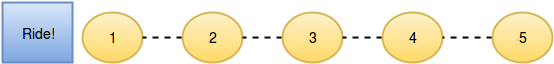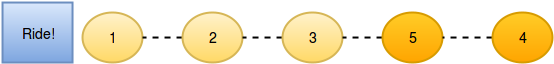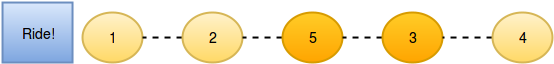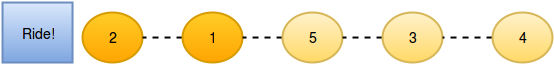New Year Chaos¶

1、题目描述¶

It's New Year's Day and everyone's in line for the Wonderland rollercoaster ride! There are a number of people queued up, and each person wears a sticker indicating their initial position in the queue. Initial positions increment by $1$ from $1$ at the front of the line to $n$ at the back.

Any person in the queue can bribe the person directly in front of them to swap positions. If two people swap positions, they still wear the same sticker denoting their original places in line. One person can bribe at most two others. For example, if $n=8$ and $Person5$ bribes $Person4$ , the queue will look like this:$[1,2,3,5,4,6,7,8]$ .

Fascinated by this chaotic queue, you decide you must know the minimum number of bribes that took place to get the queue into its current state!

Function Description

Complete the function minimumBribes in the editor below. It must print an integer representing the minimum number of bribes necessary, or Too chaotic if the line configuration is not possible.

minimumBribes has the following parameter(s):

• q: an array of integers

Input Format

The first line contains an integer , the number of test cases.

Each of the next pairs of lines are as follows:

• The first line contains an integer , the number of people in the queue
• The second line has space-separated integers describing the final state of the queue.

Constraints

• $1 \le t \le 10$
• $1 \le n \le 10^{5}$

For $60\%$ score $1 \le n \le10^{3}$ For $100\%$score $1 \le n \le 10^{5}$

Output Format

Print an integer denoting the minimum number of bribes needed to get the queue into its final state. Print Too chaotic if the state is invalid, i.e. it requires a person to have bribed more than $2$ people.

Sample Input

2
5
2 1 5 3 4
5
2 5 1 3 4

Sample Output

3
Too chaotic

Explanation

Test Case 1

The initial state:After person $5$ moves one position ahead by bribing person $4$:Now person $5$ moves another position ahead by bribing person $3$:And person $2$ moves one position ahead by bribing person $1$:So the final state is $[2,1,5,3,4]$ after three bribing operations.

Test Case 2

No person can bribe more than two people, so its not possible to achieve the input state.

2、解题思路¶

• 题目绕了几圈，实际等价于求解当前元素右面有多少个小于当前元素的值，如果超过2个，就没办法交换
• 直接利用树状数组求解，快速查询当前元素右面有多少个小的数据
#!/bin/python3

import math
import os
import random
import re
import sys

class BinaryIndexTree:
def __init__(self, nums):
"""
:type nums: List[int]
"""
self.buff =  * (nums + 1)

def update(self, i, val):
"""
:type i: int
:type val: int
:rtype: void
"""
index = i + 1
while index < len(self.buff):
self.buff[index] += val
index += index & (-index)

def getSum(self, index):
index += 1
res = 0
while index > 0:
res += self.buff[index]
index -= index & (-index)
return res

# Complete the minimumBribes function below.
def minimumBribes(q):
ans = 0
bit = BinaryIndexTree(len(q))

for index, num in enumerate(reversed(q)):

lt_num = bit.getSum(num - 1)
bit.update(num - 1, 1)
if lt_num <= 2:
ans += lt_num
else:

print("Too chaotic")
return
print(ans)

if __name__ == '__main__':
t = int(input())

for t_itr in range(t):
n = int(input())

q = list(map(int, input().rstrip().split()))

minimumBribes(q)